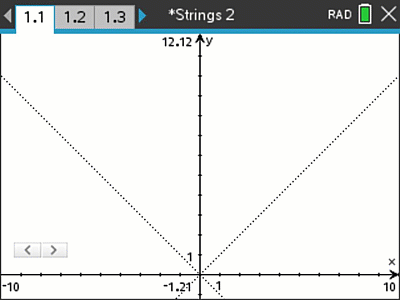# Activities

•• ##### Author60 Minutes

• ##### Device
•TI-Nspire™ CX CAS
• ##### Software

TI-Nspire™ CAS

5.0

## String Graphs Part2#### Activity Overview

This is part two of a four part series. In this activity students are given two points that lie on a straight line, they determine the equation for each line that eventually forms a curved envelope that forms a parabola. In the second half of this activity students learn to use a parameter so that the family of graphs can be generated using a single equation.

#### Objectives

Students determine the equation to a straight line given two points. In this activity they use a less familiar approach but more efficient approach by using the translational form of a straight line. This form is very quick to use and makes the identification of the subsequent patterns significantly easier. The Computer Algebra System in the calculator automatically converts the format to the more familiar y = mx + c.